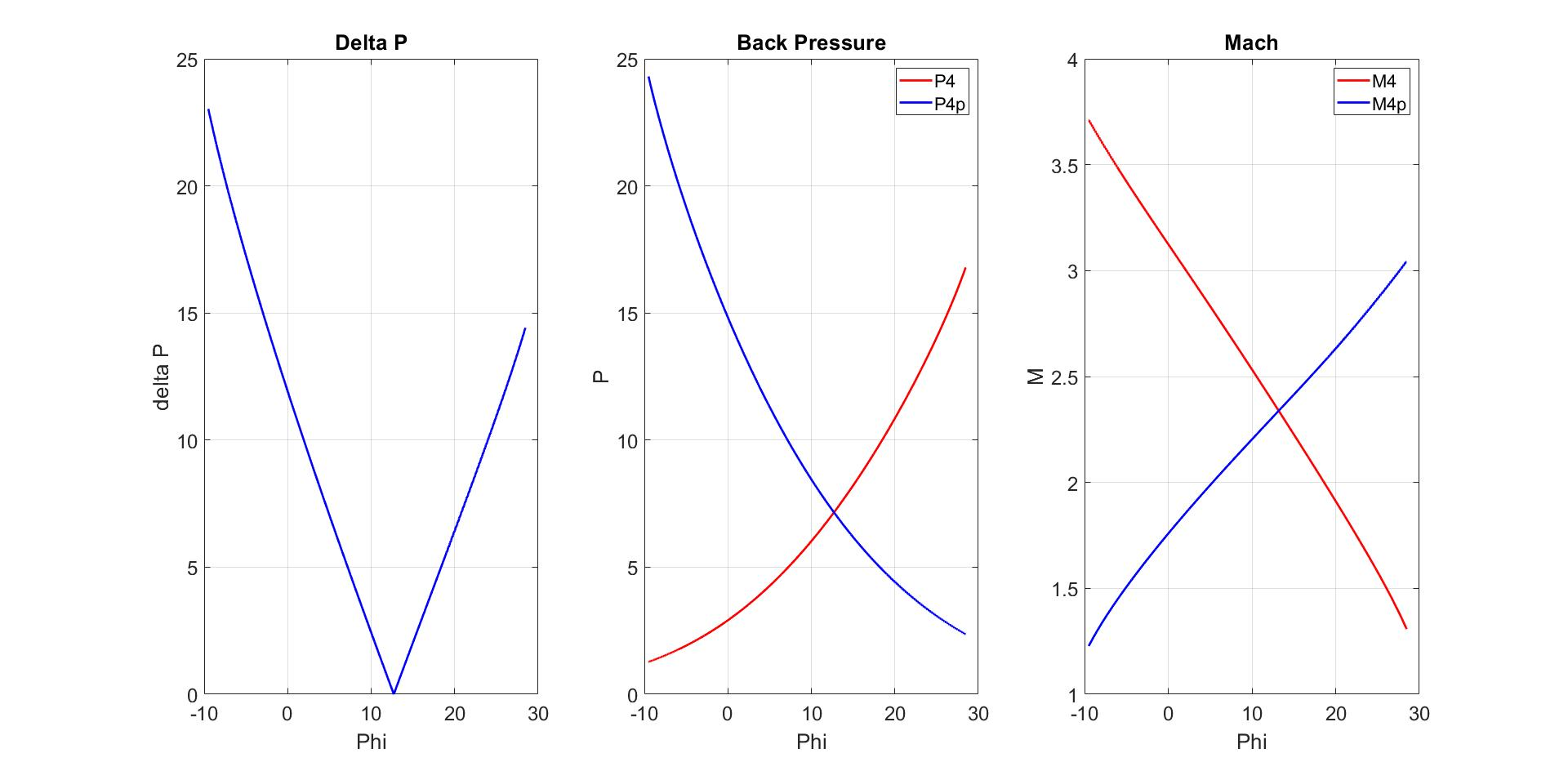## Determination the direction of the slip line

Version 1.0.0 (34 KB) by
The basic logic in this method is to determine a random initial slip line angle

Updated 7 Jul 2022

• When you run the MATLAB code, it will show you the results of the owner of this assignment. If you want to get results with any input, turn the ‘initial data’ part to comment and uncomment ‘any data’ part. It will ask you for three inputs and these are Mach number, 𝜃1 and 𝜃2 respectively.
• Two approaches are required to continue the solution in the 4 and 4′:
• - The pressures in the two zones should be the same. Otherwise the slip line is bent.
• - Although the flow velocities in the two regions are different from each other, their directions should be the same. Instead of an analytical solution, the iteration method is used. The basic logic in this method is to determine a random initial slip line angle and take the slip line angle corresponding to the value at the point where the pressure difference in the two regions is closest to zero using the iteration method.### Cite As

Anil Taha Balkir (2023). Determination the direction of the slip line (https://www.mathworks.com/matlabcentral/fileexchange/114715-determination-the-direction-of-the-slip-line), MATLAB Central File Exchange. Retrieved .

##### MATLAB Release Compatibility
Created with R2020a
Compatible with any release
##### Platform Compatibility
Windows macOS Linux